# Spatial Enhancement

## Producer Field Guide

HGD_Product
Producer Field Guide
HGD_Portfolio_Suite
Producer

While radiometric enhancements operate on each pixel individually, spatial enhancement modifies pixel values based on the values of surrounding pixels. Spatial enhancement deals largely with spatial frequency, which is the difference between the highest and lowest values of a contiguous set of pixels. Jensen (Jensen, 1986) defines spatial frequency as "the number of changes in brightness value per unit distance for any particular part of an image."

Consider the examples in the figure below:

• zero spatial frequency—a flat image, in which every pixel has the same value
• low spatial frequency—an image consisting of a smoothly varying gray scale
• highest spatial frequency—an image consisting of a checkerboard of black and white pixels

Spatial Frequencies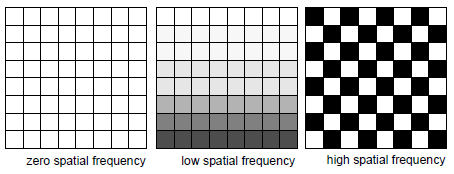This section contains a description of the following:

• Convolution, Crisp, and Adaptive filtering
• Resolution merging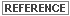See Radar Imagery Enhancement for a discussion of Edge Detection and Texture Analysis. These spatial enhancement techniques can be applied to any type of data.

Convolution Filtering

Convolution filtering is the process of averaging small sets of pixels across an image. Convolution filtering is used to change the spatial frequency characteristics of an image (Jensen, 1996).

A convolution kernel is a matrix of numbers that is used to average the value of each pixel with the values of surrounding pixels in a particular way. The numbers in the matrix serve to weight this average toward particular pixels. These numbers are often called coefficients, because they are used as such in the mathematical equations.

In ERDAS IMAGINE, you can apply convolution filtering to an image using any of these methods:

• Filtering dialog in the respective multispectral or panchromatic image type option
• Convolution function in Spatial Resolution option
• Spatial Resolution Non-directional Edge enhancement function
• Convolve function in Model Maker

Filtering is a broad term, which refers to the altering of spatial or spectral features for image enhancement (Jensen, 1996). Convolution filtering is one method of spatial filtering. Some texts may use the terms synonymously.

Convolution Example

To understand how one pixel is convolved, imagine that the convolution kernel is overlaid on the data file values of the image (in one band), so that the pixel to be convolved is in the center of the window.

Applying a Convolution Kernel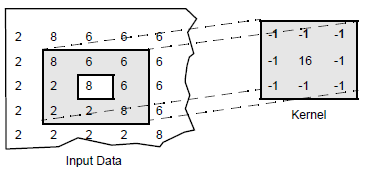The figure above shows a 3 × 3 convolution kernel being applied to the pixel in the third column, third row of the sample data (the pixel that corresponds to the center of the kernel).

To compute the output value for this pixel, each value in the convolution kernel is multiplied by the image pixel value that corresponds to it. These products are summed, and the total is divided by the sum of the values in the kernel, as shown here:

`integer [(-1 × 8) + (-1 × 6) + (-1 × 6) + (-1 × 2) + (16 × 8) + (-1 × 6) + (-1 × 2) + (-1 × 2) + (-1 × 8) ÷ (-1 + -1 + -1 + -1 + 16 + -1 + -1 + -1 + -1)]`
`= int [(128-40) / (16-8)]`
`= int (88 / 8) = int (11) = 11`

In order to convolve the pixels at the edges of an image, pseudo data must be generated in order to provide values on which the kernel can operate. In the example below, the pseudo data are derived by reflection. This means the top row is duplicated above the first data row and the left column is duplicated left of the first data column. If a second row or column is needed (for a 5 × 5 kernel for example), the second data row or column is copied above or left of the first copy and so on. An alternative to reflection is to create background value (usually zero) pseudo data; this is called Fill.

When the pixels in this example image are convolved, output values cannot be calculated for the last row and column; here we have used ?s to show the unknown values. In practice, the last row and column of an image are either reflected or filled just like the first row and column.

Output Values for Convolution Kernel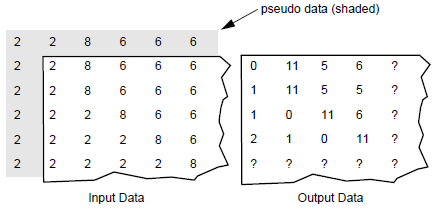The kernel used in this example is a high frequency kernel, as explained below. It is important to note that the relatively lower values become lower, and the higher values become higher, thus increasing the spatial frequency of the image.

Convolution Formula

The following formula is used to derive an output data file value for the pixel being convolved (in the center):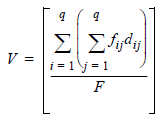Where:

fij = coefficient of a convolution kernel at position i,j (in the kernel)

dij = data value of the pixel that corresponds to fij

q = dimension of the kernel, assuming a square kernel (if q = 3, the kernel is 3 × 3)

F = either the sum of the coefficients of the kernel, or 1 if the sum of coefficients is 0

V = output pixel value

In cases where V is less than 0, V is clipped to 0.

Source: Modified from Jensen, 1996; Schowengerdt, 1983

The sum of the coefficients (F) is used as the denominator of the equation above, so that the output values are in relatively the same range as the input values. Since F cannot equal zero (division by zero is not defined), F is set to 1 if the sum is zero.

Zero-Sum Kernels

Zero-sum kernels are kernels in which the sum of all coefficients in the kernel equals zero. When a zero-sum kernel is used, then the sum of the coefficients is not used in the convolution equation, as above. In this case, no division is performed (F = 1), since division by zero is not defined.

This generally causes the output values to be:

• zero in areas where all input values are equal (no edges)
• low in areas of low spatial frequency
• extreme in areas of high spatial frequency (high values become much higher, low values become much lower)

Therefore, a zero-sum kernel is an edge detector, which usually smooths out or zeros out areas of low spatial frequency and creates a sharp contrast where spatial frequency is high, which is at the edges between homogeneous (homogeneity is low spatial frequency) groups of pixels. The resulting image often consists of only edges and zeros.

Zero-sum kernels can be biased to detect edges in a particular direction. For example, this 3 × 3 kernel is biased to the south (Jensen, 1996).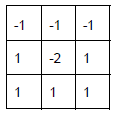See Edge Detection for more detailed information.

High-Frequency Kernels

A high-frequency kernel, or high-pass kernel, has the effect of increasing spatial frequency.

High-frequency kernels serve as edge enhancers, since they bring out the edges between homogeneous groups of pixels. Unlike edge detectors (such as zero-sum kernels), they highlight edges and do not necessarily eliminate other features.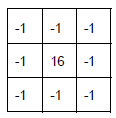When this kernel is used on a set of pixels in which a relatively low value is surrounded by higher values, like the following: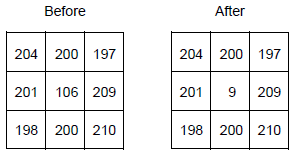Then the low value gets lower. Inversely, when the kernel is used on a set of pixels in which a relatively high value is surrounded by lower values, like the following: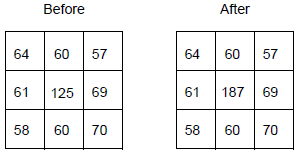Then the high value becomes higher. In either case, spatial frequency is increased by this kernel.

Low-Frequency Kernels

Below is an example of a low-frequency kernel, or low-pass kernel, which decreases spatial frequency.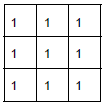This kernel simply averages the values of the pixels, causing them to be more homogeneous. The resulting image looks either more smooth or more blurred.For information on applying filters to thematic layers, see Geographic Information Systems.

Crisp

Crisp filter sharpens the overall scene luminance without distorting the interband variance content of the image. This is a useful enhancement if the image is blurred due to atmospheric haze, rapid sensor motion, or a broad point spread function of the sensor.

The algorithm used for this function is:

1) Calculate principal components of multiband input image.

2) Convolve PC-1 with summary filter.

3) Retransform to RGB space.

The logic of the algorithm is that the first principal component (PC-1) of an image is assumed to contain the overall scene luminance. The other PCs represent intra-scene variance. Thus, you can sharpen only PC-1 and then reverse the principal components calculation to reconstruct the original image. Luminance is sharpened, but variance is retained.

Resolution Merge

The resolution of a specific sensor can refer to radiometric, spatial, spectral, or temporal resolution.See Raster Data for a full description of resolution types.

Landsat TM sensors have seven bands with a spatial resolution of 28.5 m. SPOT panchromatic has one broad band with very good spatial resolution—10 m. Combining these two images to yield a seven-band data set with 10 m resolution provides the best characteristics of both sensors.

A number of models have been suggested to achieve this image merge. Welch and Ehlers (Welch and Ehlers, 1987) used forward-reverse RGB to IHS transforms, replacing I (from transformed TM data) with the SPOT panchromatic image. However, this technique is limited to three bands (R, G, B).

Chavez (Chavez et al, 1991), among others, uses the forward-reverse principal components transforms with the SPOT image, replacing PC-1.

In the above two techniques, it is assumed that the intensity component (PC-1 or I) is spectrally equivalent to the SPOT panchromatic image, and that all the spectral information is contained in the other PCs or in H and S. Since SPOT data do not cover the full spectral range that TM data do, this assumption does not strictly hold. It is unacceptable to resample the thermal band (TM6) based on the visible (SPOT panchromatic) image.

Another technique (Schowengerdt, 1980) combines a high frequency image derived from the high spatial resolution data (that is, SPOT panchromatic) additively with the high spectral resolution Landsat TM image.

Resolution Merge function has two different options for resampling low spatial resolution data to a higher spatial resolution while retaining spectral information:

• forward-reverse principal components transform
• multiplicative

Principal Components Merge

Because a major goal of this merge is to retain the spectral information of the six TM bands 1 - 5, 7), this algorithm is mathematically rigorous. It is assumed that:

• PC-1 contains only overall scene luminance; all interband variation is contained in the other 5 PCs
• Scene luminance in the SWIR bands is identical to visible scene luminance.\

With the above assumptions, the forward transform into PCs is made. PC-1 is removed and its numerical range (min to max) is determined. The high spatial resolution image is then remapped so that its histogram shape is kept constant, but it is in the same numerical range as PC-1. It is then substituted for PC-1 and the reverse transform is applied. This remapping is done so that the mathematics of the reverse transform do not distort the thematic information (Welch and Ehlers, 1987).

Multiplicative

The second technique uses a simple multiplicative algorithm: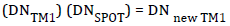The algorithm is derived from the four component technique of Crippen (Crippen, 1989a). In this paper, it is argued that of the four possible arithmetic methods to incorporate an intensity image into a chromatic image (addition, subtraction, division, and multiplication), only multiplication is unlikely to distort the color.

However, in his study Crippen first removed the intensity component via band ratios, spectral indices, or PC transform. The algorithm shown above operates on the original image. The result is an increased presence of the intensity component. For many applications, this is desirable. People involved in urban or suburban studies, city planning, and utilities routing often want roads and cultural features (which tend toward high reflection) to be pronounced in the image.

Brovey Transform

In Brovey Transform method, three bands are used according to the following formula: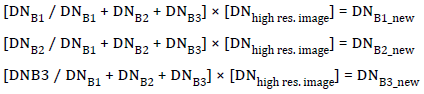Where:

B(n) = band (number)

Brovey Transform was developed to visually increase contrast in the low and high ends of an image’s histogram (that is, to provide contrast in shadows, water and high reflectance areas such as urban features). Consequently, the Brovey Transform should not be used if preserving the original scene radiometry is important. However, it is good for producing RGB images with a higher degree of contrast in the low and high ends of the image histogram and for producing visually appealing images.

Since the Brovey Transform is intended to produce RGB images, only three bands at a time should be merged from the input multispectral scene, such as bands 3, 2, 1 from a SPOT or Landsat TM image or 4, 3, 2 from a Landsat TM image. The resulting merged image should then be displayed with bands 1, 2, 3 to RGB.

Contrast enhancement (image stretching) is a widely applicable standard image processing technique. However, even adjustable stretches like the piecewise linear stretch act on the scene globally. There are many circumstances where this is not the optimum approach. For example, coastal studies where much of the water detail is spread through a very low DN range and the land detail is spread through a much higher DN range would be such a circumstance. In these cases, a filter that adapts the stretch to the region of interest (the area within the moving window) would produce a better enhancement. Adaptive filters attempt to achieve this (Fahnestock and Schowengerdt, 1983; Peli and Lim, 1982; Schwartz and Soha, 1977).

Scenes to be adaptively filtered can be divided into three broad and overlapping categories:

• Undegraded—these scenes have good and uniform illumination overall. Given a choice, these are the scenes one would prefer to obtain from imagery sources such as Space Imaging or SPOT.
• Low luminance—these scenes have an overall or regional less than optimum intensity. An underexposed photograph (scanned) or shadowed areas would be in this category. These scenes need an increase in both contrast and overall scene luminance.
• High luminance—these scenes are characterized by overall excessively high DN values. Examples of such circumstances would be an over-exposed (scanned) photograph or a scene with a light cloud cover or haze. These scenes need a decrease in luminance and an increase in contrast.

No single filter with fixed parameters can address this wide variety of conditions. In addition, multiband images may require different parameters for each band. Without the use of adaptive filters, the different bands would have to be separated into one-band files, enhanced, and then recombined.

For this function, the image is separated into high and low frequency component images. The low frequency image is considered to be overall scene luminance. These two component parts are then recombined in various relative amounts using multipliers derived from LUTs. These LUTs are driven by the overall scene luminance: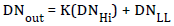Where:

K = user-selected contrast multiplier

Hi = high luminance (derives from the LUT)

LL = local luminance (derives from the LUT)

Local Luminance Intercept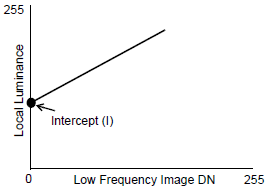The figure above shows the local luminance intercept, which is the output luminance value that an input luminance value of 0 would be assigned.